Centrifugal Punp | Minimum Starting Speed Of Centrifugal Pump

MINIMUM STARTING SPEED OF CENTRIFUGAL PUMP

To get the minimum starting speed of a centrifugal pump, it is notice that if the pressure rise in the Impeller is more than or equal to manometric head (Hm) then the centrifugal pump will start to deliver water. Otherwise the pump will not discharge any water, the impeller is rotating. If the impeller is rotating, the water in contact with the Impeller is also rotating. This is called forced vortex. In this case, the centrifugal head or head due to pressure rise in the impeller.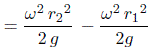Where, ωr2 = Tangential velocity of impeller at outlet = u2, and

Where, ωr1 = Tangential velocity of impeller at outlet = u1, and

So, Head due to pressure rise in impeller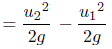The flow of water will commence only if

Head due to pressure rise in impeller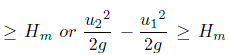For minimum speed, we must have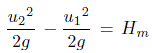But, we already know the equation, (To know this equation, read efficiency of a centrifugal pump)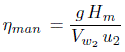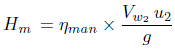Substituting this value of Hm in previous equation, we get,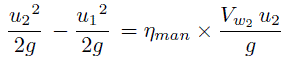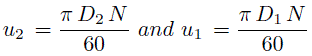Substituting the values of u2 and u1 in previous equation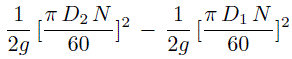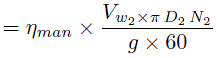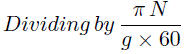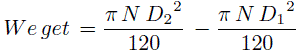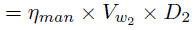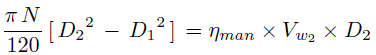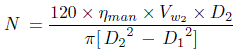N shows the minimum starting speed of a centrifugal pump.